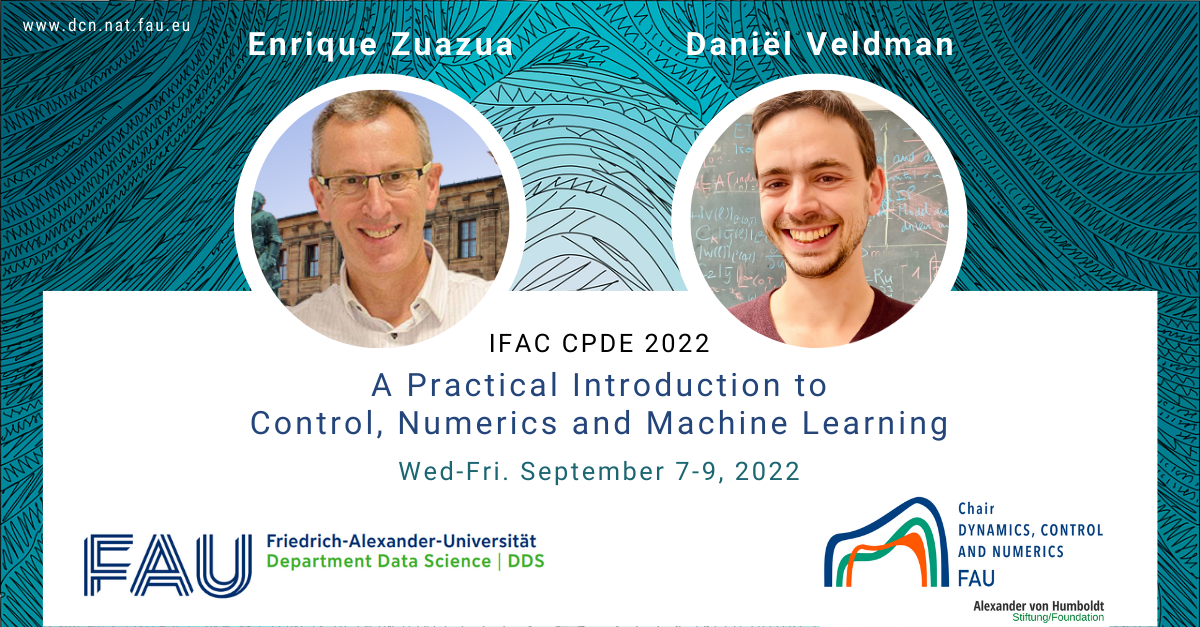# Course: A Practical Introduction to Control, Numerics, and Machine Learning (IFAC CPDE 2022)#### Practical course: Modeling, simulation, optimization

FAU DCN-AvH. Friedrich-Alexander Universität Erlangen-Nürnberg (Germany)
Period: Summer 2022 (IFAC CPDE 2022 Course)

_
This course gives a general introduction and some recent developments on the interface between Control, Numerics, and Machine Learning (Supervised Learning and Universal Approximation).

The first part of the course is a general introduction to important ideas in control theory, with an emphasis on adjoint calculus and Pontryagin’s maximum principle, which is of great theoretical interest but is also of crucial importance for the efficient numerical computation of optimal controls. This importance is illustrated by an industrial application from precision engineering, in which a thermomechanical model needs to be controlled with nanometer accuracy.

On the second day, we approach Supervised Learning from a dynamical systems perspective. We show that the training of Deep Residual Neural Networks (ResNets) and their continuous time counterparts (Neural ODEs) have close connections with classical (optimal) control theory and that these similarities are also visible in the numerics. It is demonstrated how these ideas lead to efficient numerical algorithms for Supervised Learning with deep ResNets (with an emphasis on classification problems). We also discuss the simultaneous or ensemble controllability property of ResNets, which shows that Deep ResNets possess the Universal Approximation property, a property that linear control systems do not have.

The third day is devoted to efficient algorithms to train neural networks, with an emphasis on stochastic algorithms like Stochastic Gradient Descent and the Random Batch Method.

Every day is concluded by a practical session in which the theory discussed that day is applied and implemented in Matlab. A basic working knowledge of Matlab (or similar software) is therefore recommended.

You can find the course materials below.